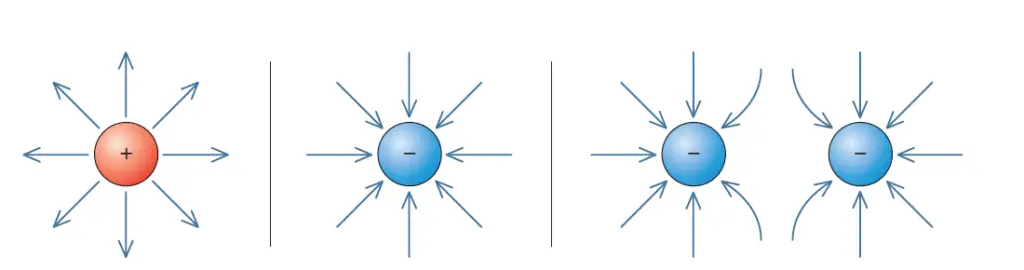# Rules to follow when you draw electric field lines around a point charge

For many applications, a much simple method is used to represent electric fields. Instead of drawing an infinite number of vector arrows, we can draw lines, called electric field lines, to represent the electric field.

While drawing the Field lines it can be monitored so that exactly one field line goes through any given point within the field, and the tangent to the field line at the point is in the direction of the electric field vector at that point.

You can give the field lines a direction such that the direction of the field line through a given point agrees with the direction of the electric field at that point.

Use the following rules when you draw electric field lines around a point charge:

• Electric field lines due to a positive source charge start from the charge and extend radially away from the charge to infinity.
• Electric field lines due to a negative source charge come from infinity radially into and terminate at the negative source charge.
• The density of lines represents the magnitude of the electric field. In other words, the more closely spaced and the greater the number of lines, the stronger is the electric field.

Figure 1 below shows how to draw electric field lines around point charges (single positive charge, single negative charge, two negative charges).Figure 1 shows how to draw electric field lines around point charges (single positive charge, single negative charge, two negative charges).

Rarely is the electric field at a point in space influenced by a single charge. Often, you need to determine the electric field for a complicated arrangement of charges. Electric field lines can be used to display these electric fields.